SSC CGL Previous Year Questions: Mensuration - 4

# SSC CGL Previous Year Questions: Mensuration - 4 - SSC CGL

Test Description

## 37 Questions MCQ Test SSC CGL (Tier - 1) - Previous Year Papers (Topic Wise) - SSC CGL Previous Year Questions: Mensuration - 4

SSC CGL Previous Year Questions: Mensuration - 4 for SSC CGL 2023 is part of SSC CGL (Tier - 1) - Previous Year Papers (Topic Wise) preparation. The SSC CGL Previous Year Questions: Mensuration - 4 questions and answers have been prepared according to the SSC CGL exam syllabus.The SSC CGL Previous Year Questions: Mensuration - 4 MCQs are made for SSC CGL 2023 Exam. Find important definitions, questions, notes, meanings, examples, exercises, MCQs and online tests for SSC CGL Previous Year Questions: Mensuration - 4 below.
Solutions of SSC CGL Previous Year Questions: Mensuration - 4 questions in English are available as part of our SSC CGL (Tier - 1) - Previous Year Papers (Topic Wise) for SSC CGL & SSC CGL Previous Year Questions: Mensuration - 4 solutions in Hindi for SSC CGL (Tier - 1) - Previous Year Papers (Topic Wise) course. Download more important topics, notes, lectures and mock test series for SSC CGL Exam by signing up for free. Attempt SSC CGL Previous Year Questions: Mensuration - 4 | 37 questions in 37 minutes | Mock test for SSC CGL preparation | Free important questions MCQ to study SSC CGL (Tier - 1) - Previous Year Papers (Topic Wise) for SSC CGL Exam | Download free PDF with solutions
 1 Crore+ students have signed up on EduRev. Have you?
SSC CGL Previous Year Questions: Mensuration - 4 - Question 1

### A circle is inscribed in an equilateral triangle and a square is inscribed in that circle. The ratio of the areas of the triangle and the square is   (SSC Multitasking 2013)

Detailed Solution for SSC CGL Previous Year Questions: Mensuration - 4 - Question 1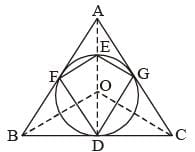In the given figure ABC is an equilateral D of side a with a circle inscribed in it and a square inscribed in the circle.
AD, BO and CO are the angle bisectors of ∠A, ∠B and ∠C and O is the centre of the circle.
We know that the angle bisector from the vertex of an equilateral triangle is the perpendicular bisector of the opposite side.
AD is the perpendicular bisector of BC.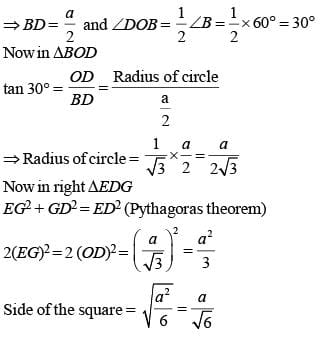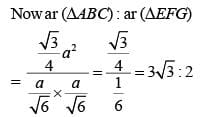SSC CGL Previous Year Questions: Mensuration - 4 - Question 2

### A circular road runs around a circular ground. If the difference between the circumferences of the outer circle and the inner circle is 66 metres, the width of the road is: (Take π = 22/7)   (SSC CGL 1st Sit.2013)

Detailed Solution for SSC CGL Previous Year Questions: Mensuration - 4 - Question 2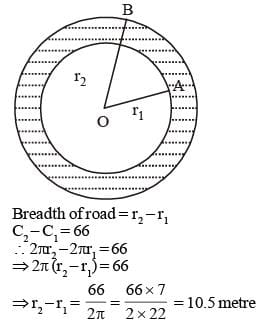SSC CGL Previous Year Questions: Mensuration - 4 - Question 3

### The perimeter of the base of a right circular cone is 8 cm. If the height of the cone is 21 cm, then its volume is:   (SSC CGL 1st Sit. 2013)

Detailed Solution for SSC CGL Previous Year Questions: Mensuration - 4 - Question 3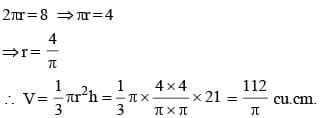SSC CGL Previous Year Questions: Mensuration - 4 - Question 4

The ratio of inradius and circumradius of a square is:    (SSC CGL 1st Sit. 2013)

Detailed Solution for SSC CGL Previous Year Questions: Mensuration - 4 - Question 4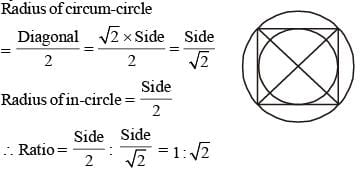SSC CGL Previous Year Questions: Mensuration - 4 - Question 5

The length and breadth of a square are increased by 30% and 20% respectively. The area of the rectangle so formed exceeds the area of the square by:    (SSC CHSL 2012)

Detailed Solution for SSC CGL Previous Year Questions: Mensuration - 4 - Question 5

Increased area of rectangle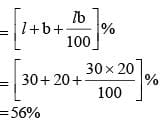Therefore, area of rectangle exceeds the area of square by 56%.

SSC CGL Previous Year Questions: Mensuration - 4 - Question 6

A metal pipe of negligible thickness has radius 21 cm and length 90 cm. The outer curved surface area of the pipe in square cm is   (SSC CGL 2nd Sit. 2012)

Detailed Solution for SSC CGL Previous Year Questions: Mensuration - 4 - Question 6

Curved surface area of cylinder = 2πrh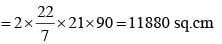SSC CGL Previous Year Questions: Mensuration - 4 - Question 7

A rectangular garden is 100 m × 80 m. There is a path along the garden and just outside it. Width of path is 10 m. The area of the path is    (SSC CGL 1st Sit. 2012)

Detailed Solution for SSC CGL Previous Year Questions: Mensuration - 4 - Question 7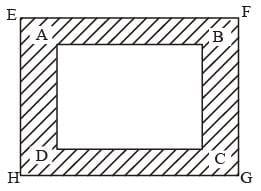= (100 + 2 × 10) (80 + 2 × 10) – 100 × 80
=  120 × 100 – 8000
= 4000 sq. metre

SSC CGL Previous Year Questions: Mensuration - 4 - Question 8

The area of the largest triangle that can be inscribed in a semicircle of radius x in square units is:   (SSC CGL 2nd Sit. 2012)

Detailed Solution for SSC CGL Previous Year Questions: Mensuration - 4 - Question 8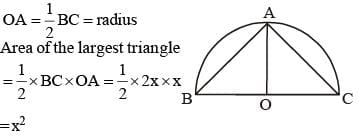SSC CGL Previous Year Questions: Mensuration - 4 - Question 9

The capacities of two hemispherical vessels are 6.4 litres and 21.6 litres. The ratio of their inner radii is    (SSC CGL 1st Sit. 2012)

Detailed Solution for SSC CGL Previous Year Questions: Mensuration - 4 - Question 9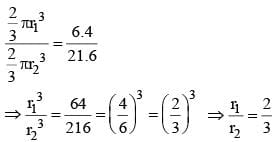SSC CGL Previous Year Questions: Mensuration - 4 - Question 10

The perimeter of a semi-circular area is 18 cm, then the radius is: (using π = 22/7)    (SSC CHSL 2012)

Detailed Solution for SSC CGL Previous Year Questions: Mensuration - 4 - Question 10

Perimeter of a semicircular area = 18 cm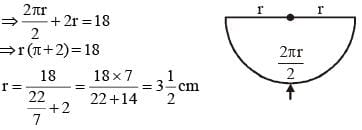SSC CGL Previous Year Questions: Mensuration - 4 - Question 11

The ratio of the edges of rectangular parallelopiped is 1 : 2 : 3 and its volume is 1296 cubic cm. The area of the whole surface in sq. cm is:    (SSC CHSL 2012)

Detailed Solution for SSC CGL Previous Year Questions: Mensuration - 4 - Question 11

Volume of rectangular parallelopiped = 1296
Ratio of edges = 1 : 2 : 3
∴ x, 2x and 3x are length, breadth and height of parallelopiped respectively.
x × 2x × 3x = 1296
⇒ 6x3 = 1296 ⇒ x3 = 216 ⇒ x = ∛216 = 6
Length = 6, Breadth = 12, Height = 18
Required surface area = 2 (lb + bh + hl)
= 2 (6 × 12 + 12 × 18 + 18 × 6) = 792 sq.cm

SSC CGL Previous Year Questions: Mensuration - 4 - Question 12

Diagonal of a cube is 6√3 cm. Ratio of its total surface area and volume (numerically) is:   (SSC CHSL 2012)

Detailed Solution for SSC CGL Previous Year Questions: Mensuration - 4 - Question 12

Diagonal of a cube = 6√3
√3 × side = 6√3
∴ Side of a cube = 6
Surface area of cube = 6 × (side)2 = 6 × 62
Volume of cube = (side)3 = (6)3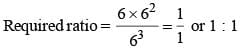SSC CGL Previous Year Questions: Mensuration - 4 - Question 13

The circumference of the base of a 16 cm height solid cone is 33 cm. What is the volume of the cone in cm3?   (SSC CHSL 2012)

Detailed Solution for SSC CGL Previous Year Questions: Mensuration - 4 - Question 13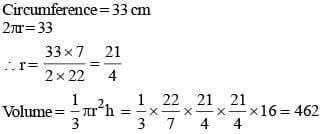SSC CGL Previous Year Questions: Mensuration - 4 - Question 14

The length of a minute hand of a clock is 7 cm. The area swept by the minute hand in 30 minutes is:   (SSC CHSL 2012)

Detailed Solution for SSC CGL Previous Year Questions: Mensuration - 4 - Question 14

Angle made by clock in 30 minutes = 180°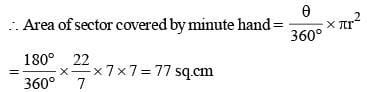SSC CGL Previous Year Questions: Mensuration - 4 - Question 15

The volume of a cubical box is 3.375 cubic meters. The length of edge of the box is:  (SSC CHSL 2012)

Detailed Solution for SSC CGL Previous Year Questions: Mensuration - 4 - Question 15

Volume of cubical box = 3.375 m3
Length of edge of the box = ∛3.375 = 1.5 m

SSC CGL Previous Year Questions: Mensuration - 4 - Question 16

If the numerical value of the volume of a right circular cylinder and its curved surface area are equal, then its radius is    (SSC Sub. Ins. 2012)

Detailed Solution for SSC CGL Previous Year Questions: Mensuration - 4 - Question 16

Volume of right circular cylinder = Curved surface area of cylinder
⇒ πr2h = 2πrh   [where, r → radius; h → height] :
⇒ r = 2 units

SSC CGL Previous Year Questions: Mensuration - 4 - Question 17

Volume of a right circular cone is numerically equal to its slant surface area. Then value of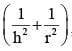, where h and r are height and radius of the cone respectively, is    (SSC Sub. Ins. 2012)

Detailed Solution for SSC CGL Previous Year Questions: Mensuration - 4 - Question 17

According to condition given
Volume of right circular cone = Slant surface area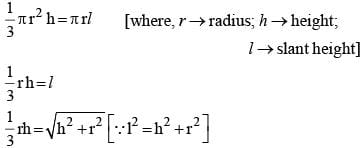Squaring on both sides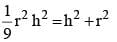Dividing equation by r2h2 on both sides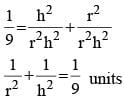SSC CGL Previous Year Questions: Mensuration - 4 - Question 18

The area of a semi-circular field is 308 sq. m; then taking π = 22/7, the length of the railing to surround it has to be   (SSC Sub. Ins. 2012)

Detailed Solution for SSC CGL Previous Year Questions: Mensuration - 4 - Question 18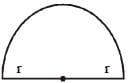Length of railing to surround = Length of Are + Length of diameter Area of semicircular = 308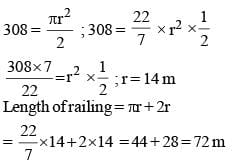SSC CGL Previous Year Questions: Mensuration - 4 - Question 19

ABCDEF is a regular hexagon of side 2 feet. The area, in square feet of the rectangle BCEF is    (SSC Sub. Ins. 2012)

Detailed Solution for SSC CGL Previous Year Questions: Mensuration - 4 - Question 19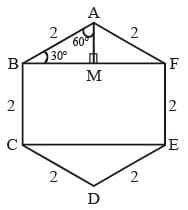Given BC & EF are each 2 feet. Since area of rectangle is length × width.
To find out BF or CE, Take DABF. It has two equal sides (AB = AF), so the perpendicular from A to line BF divides ABF into two congruent Ds.
So, each of the two triangles is 30°-60°-90° right angle D with hypotenuse 2.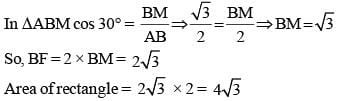SSC CGL Previous Year Questions: Mensuration - 4 - Question 20

The base of a triangle is 2 cm more than twice its altitude. If the area is 12 sq. cm, its altitude will be    (SSC Sub. Ins. 2012)

Detailed Solution for SSC CGL Previous Year Questions: Mensuration - 4 - Question 20

Base = 2 + 2 × altitude
Let altitude be A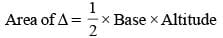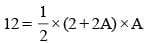12 = A × (1 + A) ; 12 = A + A2
A2 + A – 12 = 0 ; (A – 3) (A + 4) = 0
A = 3, A = – 4
Altitude = 3 cm

SSC CGL Previous Year Questions: Mensuration - 4 - Question 21

The sides of a triangles are in the ratio 2 : 3 : 4. the perimeter of the triangle is 18cm. The area (in cm2) of the triangle is    (SSC CGL 2nd Sit. 2011)

Detailed Solution for SSC CGL Previous Year Questions: Mensuration - 4 - Question 21

Ratio = 2 : 3 : 4 = 4 : 6 : 8
Perimeter = 18 cm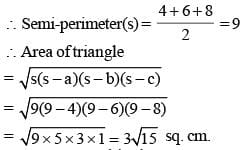SSC CGL Previous Year Questions: Mensuration - 4 - Question 22

The wheel of a motor car makes 1000 revolutions in moving 440 m. The diameter (in metre) of the wheel is    (SSC CGL 2nd Sit. 2011)

Detailed Solution for SSC CGL Previous Year Questions: Mensuration - 4 - Question 22

Distance covered by wheel in one revolution = Circumference of wheel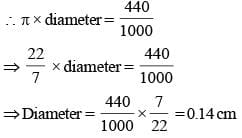SSC CGL Previous Year Questions: Mensuration - 4 - Question 23

Water is flowing at the rate of 5 km/h through a pipe of diameter 14cm into a rectangular tank which is 50 m long, 44m wide. The time taken, in hours, for the rise in the level of water in the tank to be 7 cm is    (SSC CGL 2nd Sit. 2011)

Detailed Solution for SSC CGL Previous Year Questions: Mensuration - 4 - Question 23

Water flowed by the pipe in 1 hr. = πr2h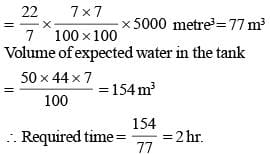SSC CGL Previous Year Questions: Mensuration - 4 - Question 24

The areas of three consecutive faces of a cuboid are 12 cm2, 20 cm2 and 15 cm2, then the volume (in cm3) of the cuboid is    (SSC CGL 2nd Sit. 2011)

Detailed Solution for SSC CGL Previous Year Questions: Mensuration - 4 - Question 24

If the length, breadth and height of the cuboid be x, y and z cm respectively, then
xy = 12; yz = 20; zx = 15
∴ x2y2z2 = 12 × 20 × 15 = 3600 cm6
∴ n = xyz = √3600 = 60 cm3

SSC CGL Previous Year Questions: Mensuration - 4 - Question 25

A copper wire is bent in the form of square with an area of 121 cm2. It the same wire is bent in the form of a circle, the radius (in cum) of the circle is (Take π = 22/7)    (SSC CGL 2nd Sit. 2011)

Detailed Solution for SSC CGL Previous Year Questions: Mensuration - 4 - Question 25

Side of square = 121 = 11 cm
∴ Length of wire = 4 × 11 = 44 cm
Circumference of circle = Length of wire
∴ 2πr = 44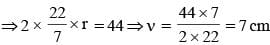SSC CGL Previous Year Questions: Mensuration - 4 - Question 26

The perimeter of a triangle is 40cm and its area is 60 cm2. If the largest side measures 17cm, then the length (in cm) of the smallest side of the triangle is    (SSC CGL 1st Sit. 2011)

Detailed Solution for SSC CGL Previous Year Questions: Mensuration - 4 - Question 26

Smallest side of the triangle = x cm (let)
∴ Second side of triangle
= 40 – 17 – x = 23 – x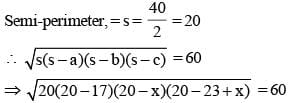⇒ (20 – x) (x – 3) = 60
⇒ 20x – 60 – x2 + 3x = 60
⇒ x2 – 23x + 120 = 0
⇒ x2 – 15x – 8x + 120 = 0
⇒ x (x – 15) – 8 (x – 15) = 0
⇒ (x – 8) (x – 15) = 0
⇒ x = 8 or 15
Since, x is the smallest side
Then, x < 23 – x. Hence, x = 8 and x ≠ 15

SSC CGL Previous Year Questions: Mensuration - 4 - Question 27

A bicycle wheel makes 5000 revolutions in moving  11 km. Then the radius of the wheel (in cm) is (Take π = 22/7)    (SSC CGL 1st Sit. 2011)

Detailed Solution for SSC CGL Previous Year Questions: Mensuration - 4 - Question 27

Distance covered by wheel in one revolution = Circumference of wheel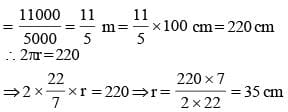SSC CGL Previous Year Questions: Mensuration - 4 - Question 28

A river 3 m deep and 40 m wide is flowing at the rate of 2 km per hour. How much water (in litres) will fall into the sea in a minute?   (SSC CGL 1st Sit. 2011)

Detailed Solution for SSC CGL Previous Year Questions: Mensuration - 4 - Question 28

Volume of water flowed in an hour = 2000 × 40 × 3 m3
= 240000 m3
∴ Volume of water flowed in 1 minute.
= 240000/60 = 4000 m3 = 4000000 litre

SSC CGL Previous Year Questions: Mensuration - 4 - Question 29

The volume (in m3) of rain water that can be collected from 1.5 hectares of ground in a rainfall of 5 cm is    (SSC CGL 1st Sit. 2011)

Detailed Solution for SSC CGL Previous Year Questions: Mensuration - 4 - Question 29

1 hectare = 10000 sq. metre
∴ Area of the ground = 15000 sq. metre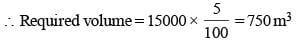SSC CGL Previous Year Questions: Mensuration - 4 - Question 30

A copper wire is bent in the shape of a square of area 81 cm2. If the same wire is bent form of a semicircle, the radius (in cm) of the semicircle is  (Take π = 22/7)   (SSC CGL 1st Sit. 2011)

Detailed Solution for SSC CGL Previous Year Questions: Mensuration - 4 - Question 30

Side of a square = √81 = 9 cm
∴ Length of the wire = 4 × 9 = 36 cm.
∴ Perimeter of semi-circle = Length of wire
⇒ (p + 2)r = 36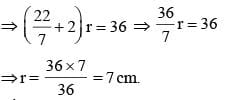SSC CGL Previous Year Questions: Mensuration - 4 - Question 31

If the length of a rectangle is increased by 10% and its breadth is decreased by 10%, the change in its area will be    (SSC CGL 2nd Sit. 2010)

Detailed Solution for SSC CGL Previous Year Questions: Mensuration - 4 - Question 31

Required change in area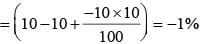Negative sign shows a decrease.

SSC CGL Previous Year Questions: Mensuration - 4 - Question 32

The ratio of the radii of two wheels is 3 : 4. The ratio of their circumferences is     (SSC CGL 2nd Sit. 2010)

Detailed Solution for SSC CGL Previous Year Questions: Mensuration - 4 - Question 32

Ratio of the circumferences = Ratio of radii = 3 : 4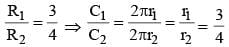SSC CGL Previous Year Questions: Mensuration - 4 - Question 33

A copper wire of length 36 m and diameter 2 mm is melted to form a sphere. The radius of the sphere (in cm) is    (SSC CGL 2nd Sit. 2010)

Detailed Solution for SSC CGL Previous Year Questions: Mensuration - 4 - Question 33

Volume of the wire = πr2h
= π × 0.1 × 0.1 × 3600 cm3  = 36p cm3
Volume of wire = vol. of sphere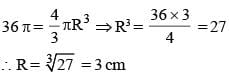SSC CGL Previous Year Questions: Mensuration - 4 - Question 34

If each side of a square is increased by 10%, its area will be increased by     (SSC CGL 2nd Sit. 2010)

Detailed Solution for SSC CGL Previous Year Questions: Mensuration - 4 - Question 34

Increase percent in area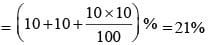SSC CGL Previous Year Questions: Mensuration - 4 - Question 35

If the circumference of a circle is decreased by 50% then the percentage of decrease in its area is   (SSC CGL 1st Sit. 2010)

Detailed Solution for SSC CGL Previous Year Questions: Mensuration - 4 - Question 35

Circumference = 2πr (one variable)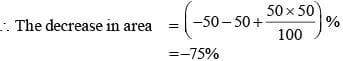SSC CGL Previous Year Questions: Mensuration - 4 - Question 36

The length (in metres) of the longest rod that can be put in a room of dimensions 10 m × 10 m × 5 m is    (SSC CGL 1st Sit. 2010)

Detailed Solution for SSC CGL Previous Year Questions: Mensuration - 4 - Question 36

Length of the longest rod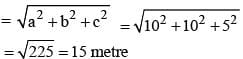SSC CGL Previous Year Questions: Mensuration - 4 - Question 37

An equilateral triangle of side 6 cm has its corners cut off to form a regular hexagon. Area (in cm2) of this regular hexagon will be    (SSC CGL 1st Sit.  2010)

Detailed Solution for SSC CGL Previous Year Questions: Mensuration - 4 - Question 37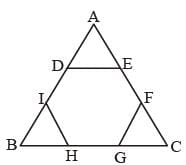Side of the regular hexagon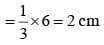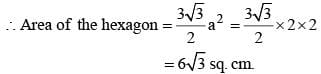## SSC CGL (Tier - 1) - Previous Year Papers (Topic Wise)

250 tests
Information about SSC CGL Previous Year Questions: Mensuration - 4 Page
In this test you can find the Exam questions for SSC CGL Previous Year Questions: Mensuration - 4 solved & explained in the simplest way possible. Besides giving Questions and answers for SSC CGL Previous Year Questions: Mensuration - 4, EduRev gives you an ample number of Online tests for practice

250 tests